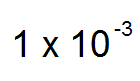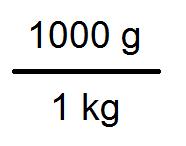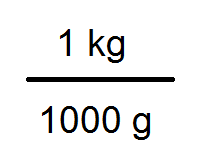# Matter & Experimental Chemistry Flashcards

Matter & Experimental Chemistry Flashcards
1/39 (missed) 0 0
Create Your Account To Continue Studying

As a member, you'll also get unlimited access to over 75,000 lessons in math, English, science, history, and more. Plus, get practice tests, quizzes, and personalized coaching to help you succeed.

Try it risk-free for 30 days. Cancel anytime.
###### Already registered? Log in here for access
9.45 x 2.00, rounded to the correct number of significant figures
18.9
Got it
33/9, rounded off to the correct number of significant figures
4
Got it
9.45 x 2, rounded off to the correct number of significant figures
20
Got it
Number of significant figures in 93
Two
Got it
Number of significant figures in 0.00900
Three
Got it
Number of significant figures in 0.009
One
Got it
Number of significant figures in 0.903
Three
Got it
Number of significant figures in 903
Three
Got it
Number of significant figures in 0.9
One
Got it
Number of significant figures in 0.900
Three
Got it
Number of significant figures in 900
One
Got it
State of matter for air
Gas
Got it
State of matter for an ice cube
Solid
Got it
State of matter for room-temperature coffee
Liquid
Got it
There are _____ millimeters per meter
1000
Got it
0.001 in scientific notation
1 x 10^-3Got it
Conversion factor for converting kilograms into grams
(1000 g) / (1 kg)Got it
Conversion factor for converting grams into kilograms
(1 kg) / (1000 g)Got it
There are _____ milliliters per liter
1000
Got it

### Ready to move on?

or choose a specific lesson: See all lessons in this chapter
39 cards in set

## Flashcard Content Overview

This flashcard set presents fundamentals for learning chemistry. You can test your memory of what metric prefixes like 'milli-' and 'centi-' mean. The cards review core principles of scientific notation and significant figures. They also offer practice problems for identifying how many significant figures are in a certain number. Students will also practice rounding calculation results based on significant figures in the problem. These flashcards also cover the meaning of various states of matter and how they are related.

Front
Back
There are _____ milliliters per liter
1000
Conversion factor for converting grams into kilograms
(1 kg) / (1000 g)Conversion factor for converting kilograms into grams
(1000 g) / (1 kg)0.001 in scientific notation
1 x 10^-3There are _____ millimeters per meter
1000
State of matter for room-temperature coffee
Liquid
State of matter for an ice cube
Solid
State of matter for air
Gas
Number of significant figures in 900
One
Number of significant figures in 0.900
Three
Number of significant figures in 0.9
One
Number of significant figures in 903
Three
Number of significant figures in 0.903
Three
Number of significant figures in 0.009
One
Number of significant figures in 0.00900
Three
Number of significant figures in 93
Two
9.45 x 2, rounded off to the correct number of significant figures
20
33/9, rounded off to the correct number of significant figures
4
9.45 x 2.00, rounded to the correct number of significant figures
18.9
32.000 / 8.00, rounded off to the correct number of significant figures
4.00
Solids can melt into this state of matter
Liquid
Liquids can freeze into this state of matter
Solid
Liquids can vaporize into this state of matter
Gas
Gases can condense into this state of matter
Liquid
There are _____ liters per milliliter
0.001
When do non-zero numbers count as significant figures

Always

When zeroes count as significant figures

When they appear between two other numbers.

When they are the last digit(s) after a decimal point.

When zeroes are not significant figures

When they appear as the last digit(s) in a number without a decimal point.

When they are leading zeroes (the first digits in a number with a decimal point).

There are _____ centimeters per meter
100
When a number is shown in scientific notation, it has _____ digits before the decimal point.
One digit
When a number in regular notation is larger than 1, and you need scientific notation, the decimal point moves _____.
Move the decimal point to the left, to convert regular notation to scientific notation.
When a number in regular notation is larger than 1, its scientific-notation integer will be _____.

The scientific-notation integer will be positive

When a number in regular notation is smaller than 1, and you need scientific notation, the decimal point moves _____.
Move the decimal point to the right, to convert regular notation to scientific notation.
When a number in regular notation is smaller than 1, its scientific-notation integer will be _____.
The scientific-notation integer will be negative.
When adding or subtracting with significant figures
Round your answer so it has as many decimal places as the problem's number with the fewest decimal places
When multiplying or dividing with significant figures
Round your answer so it has as many significant figures as the problem's number with the fewest significant figures.
State of matter with a specific shape and a specific volume
Solid
State of matter with a specific volume that flows into any shape of container.
Liquid
State of matter whose shape and volume can both change
Gas

To unlock this flashcard set you must be a Study.com Member.
Create your account

### Unlock Your Education

#### See for yourself why 30 million people use Study.com

##### Become a Study.com member and start learning now.

Already a member? Log In

Support